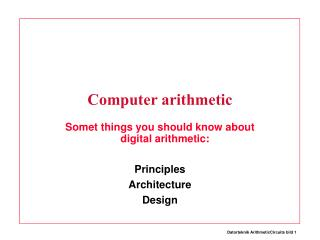Download PresentationComputer arithmetic

# Computer arithmetic - PowerPoint PPT PresentationDownload Presentation## Computer arithmetic

- - - - - - - - - - - - - - - - - - - - - - - - - - - E N D - - - - - - - - - - - - - - - - - - - - - - - - - - -
##### Presentation Transcript

1. Computer arithmetic Somet things you should know about digital arithmetic: Principles Architecture Design

2. Multiplication • Can be done in one clock cycle but: • Very slow • Needs a lot of hardware

3. Multiplication Si m n m Si n n Ci Co Ci So Co m So

4. Multiplication b3 b2 b1 b0 a0 a0b3 a0b2 a0b1 a0b0 a1 a1b3 a1b2 a1b1 a1b0 a2 a2b3 a2b2 a2b1 a2b0 a3 a3b3 a3b2 a3b1 a3b0 p7 p6 p5 p4 p3 p2 p1 p0

5. To avoid those costs: • Multiplication is usually multiple-cycle • For example: Repeated add, shift • In the MIPS: “4 - 12 cycles for mult” Databook s 3.9 Multiply instruction is not implemented in our simulator

6. Division... • is even worse.... • Multiple cycle • Repeated shift - subtract - test • Databook ... instruction uses 35 cycles Divide instruction is not implemented in our simulator

7. Division • can be done by D shifted right n bits • When D is negative: • If D is even: D Arithmetic shift right by n • If D is odd: (D + 1) Arithmetic shift right by n n D / 2

8. Example - 1 / 2 -1 arith. shift right 1: 111 -> 111 result: -1 Wrong (-1 + 1) arith. shift right 1: 000 -> 000 result: 0 OK

9. In the mips, • Multiply and divide uses special hardware (not the ALU) • and special registers “HI”, “LO” (not in our simulator)

10. Floating point? • Needs its own hardware! • Co-processor, usually a separate chip Main (integer) CPU CP1 Floating point CP0 Control

11. So the ALU does ADD SUBTRACT SIMPLE LOGIC Simple logic is fast, but add / sub is slow because of long critical path

12. Add two numbers 1100 .......................010 +.......................110 000 Carry from step n-1 3 input bits in each step Sum Cin B0 Full adder S0 A0 Cout

13. & & & The full adder A B Ci S Co 0 0 0 0 0 0 0 1 1 0 0 1 0 1 0 0 1 1 0 1 1 0 0 1 0 1 0 1 0 1 1 1 0 0 1 1 1 1 1 1 2-level logic or: A =1 B =1 S Ci Co

14. B2 B1 B29 B0 B31 B30 A2 A1 A29 A0 A31 A30 Cin S2 S1 S29 S0 S31 S30 The carry chain Cout

15. B2 B1 B29 B0 B31 B30 A2 A1 A29 A0 A31 A30 0 S2 S1 S29 S0 S31 S30 Addition

16. Subtraction A - B ? A + Neg (B) two’s complement A + Not (B) + 1 one’s complement + 1

17. B31 B31 B31 B31 B31 B31 B31 =1 =1 =1 =1 =1 =1 =1 Add and subtract A2 A1 A29 A0 A31 A30 0 -> add 1 -> sub S2 S1 S29 S0 S31 S30

18. Timing analys • There are six gates per stage* * Exor are two gate levels • There are 32 stages • The critical path are 6 * 32 gate delay! (Ripple adder) • We must break up that carry chain!

19. A =1 P B A & G B Full adder again: • S = A xor B xor Ci • Co = (A and B) or ((A xor B) and Ci) • We define • P = A xor B • G = A and B • And we get • S = P xor Ci • Co = G or (P and Ci) Computed quickly!

20. The full adder .... • Si = Pi xor Ci-1 • Ci = Gi or (Pi and Ci-1) • If we could be given all of the Ci at the same time, Si is just one more xor

21. The full adder • C0 = G0 or (P0 and Cin) • C1 = G1 or (P1 and C0) • C1 = G1 or (P1 and (G0 or (P0 and Cin)) • C1 = G1 or P1G0 or P1P0Cin • in the K:th position: • Ck = Gk or Gk-1Pk or....PkPk-1....P0Cin Wide or Wide and

22. Cin Carry generator (two level logic) Final add (exor) P / G generator (two level logic) G C 32 32 32 A 32 S P 32 32 32 B The carry lookahead adder

23. At the worst... • An N-input AND (OR) has delay lg2 (N) * 2-input delay:

24. C8 C12 C0 C4 C C C C P12,15 P4,7 G12,15 G4,7 P8,11 G8,11 P0,3 G0,3 B B C8 C0 B C0 The combination of carry lookahead and ripple carry

25. G12,15 G8,11 G0,3 G4,7 Full adder Full adder Full adder Full adder C0 C4 C8 C12 ≥1 ≥1 P12,15 P8,11 P4,7 P0,3 & & & The carry skip adder - If the full adder in step n generates a carry, it will be correct independent of carry in. - A carry generated in step n is propagated through the and / or gates, not through the adders

26. B B A A B B A A B B A A B A ≥1 The carry select adder C0 Full adder 0 Full adder 0 Full adder Full adder 0 S 1 Full adder Full adder 1 Full adder 1 S S S ≥1 & &

27. Asymptotic time and space requirements Time Space • Ripple carry O(n) O(n) • Carry lookahead O(log n) O(n log n) • Carry skip O(sqrt n) O(n) • Carry select O(sqrt n) O(n)